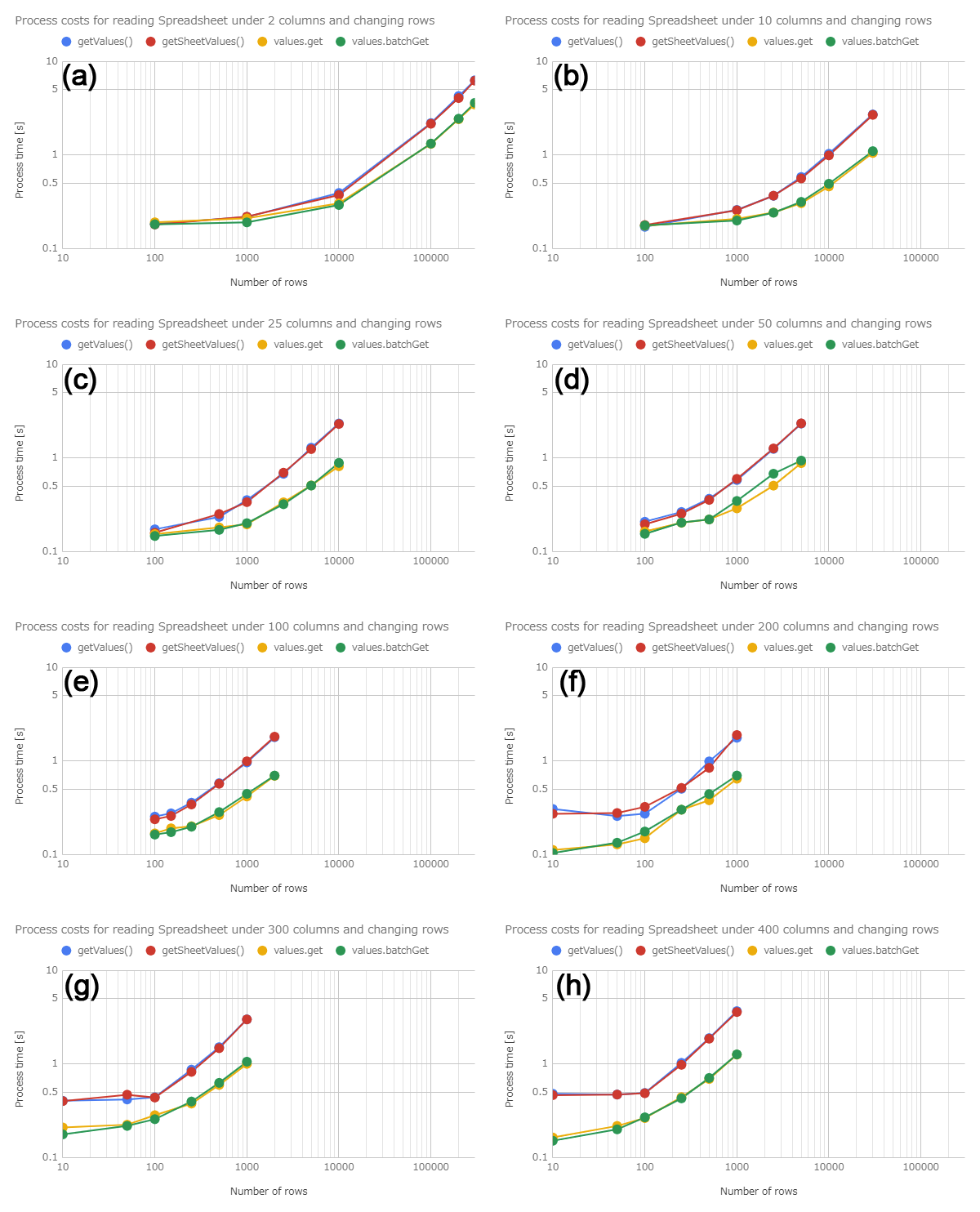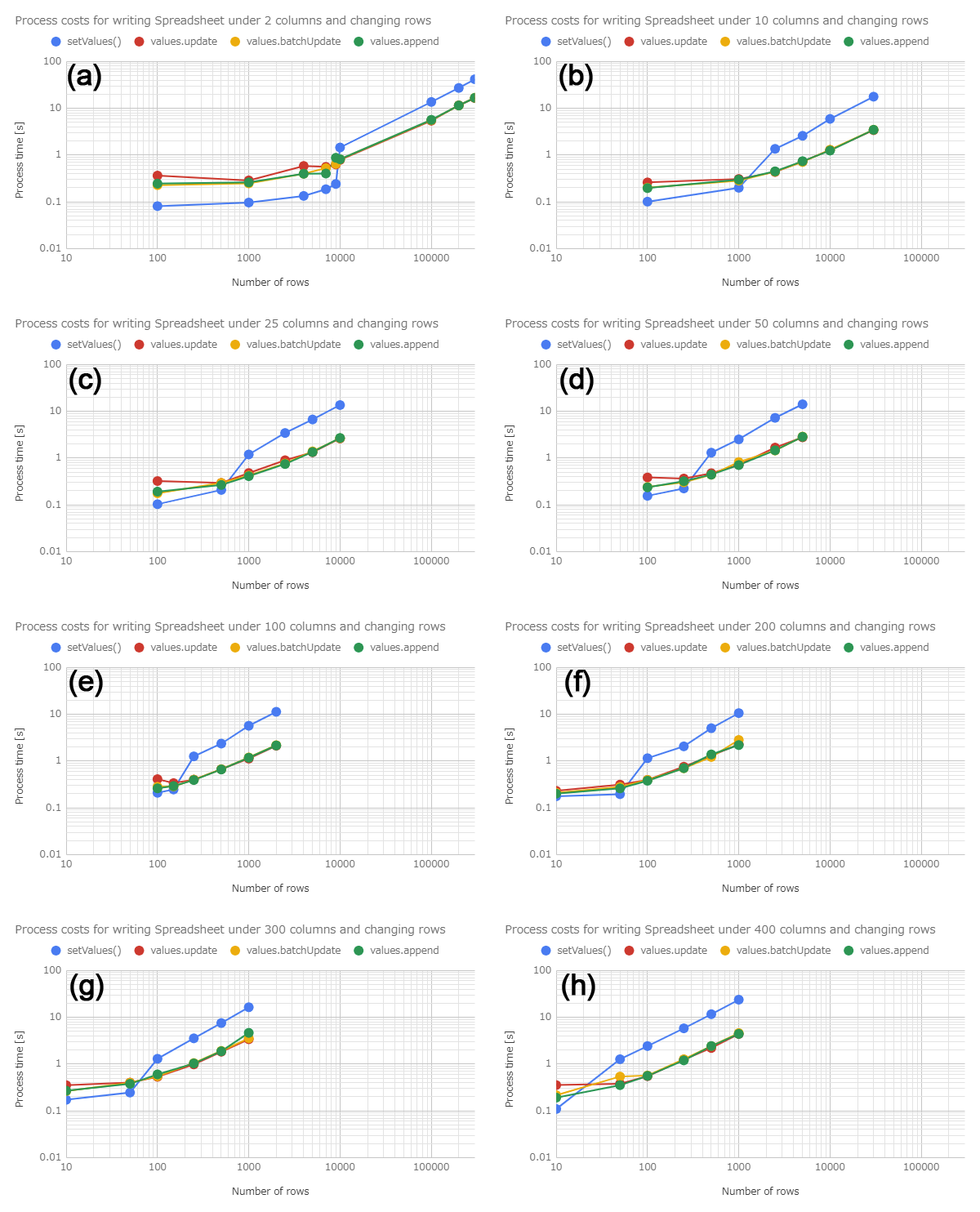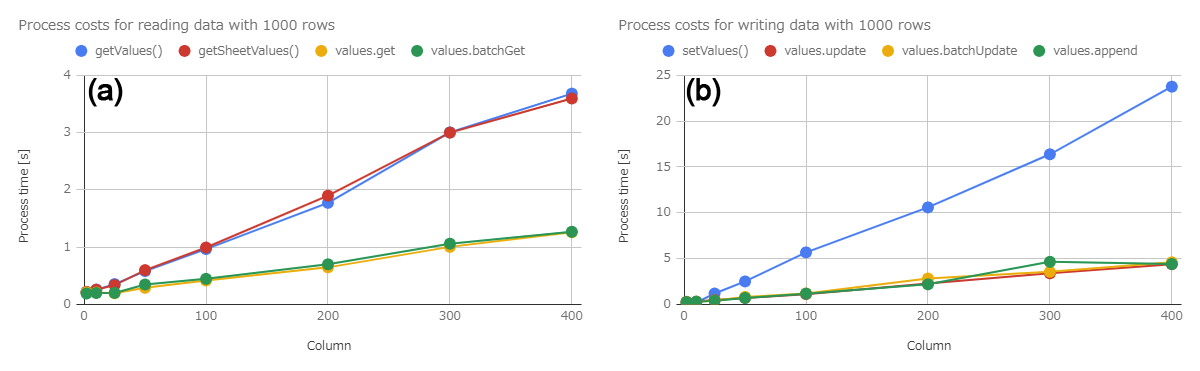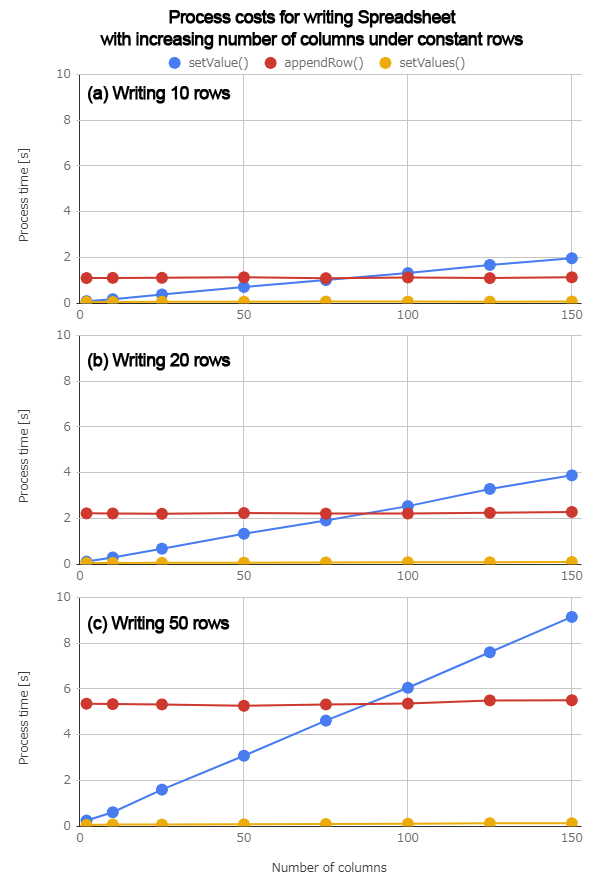Gists

October 18, 2018 Updated. In order to compare with Advanced Google Service, a result of Sheets API by UrlFetchApp was added to Appendix.

Kanshi Tanaike

# Introduction

Please be careful! This result can be only used for Google Apps Script.

There are a limit executing time for Google Apps Script (GAS). That is 6 minutes for Consumer and Google Apps free edition, and 30 minutes for G Suite and Early Access. 1 So many users always have to pay attention to reducing the process cost of scripts. So it is very important to know the process cost of various situations. I have already reported the costs for various processes as the reports. 2 In this report, the process cost for reading and writing values for Spreadsheet using GAS has been investigated.

As the result, the conclusion that the methods for reading and writing Spreadsheet of Sheets API are using the different algorithm and/or process from those of Spreadsheet service were derived.

# Experimental procedure

In order to measure the process cost for reading and writing spreadsheet, the size of values for reading and writing were variously changed. For measuring the reading cost, Spreadsheet which had the values for reading was used. For measuring the writing cost, Spreadsheet that all values were cleared was used. The methods for reading and writing which were used this report are as follows.

Tests Methods used for measuring process cost
Writing (Spreadsheet Service) setValue(), appendRow(), setValues()
Writing (Sheets API) values.update, values.batchUpdate, values.append

For the methods of Sheets API, the reason these methods are chosen is because 2 dimensional array can be used as the values. At the flow of each method, the spreadsheet is opened and is read or written. From the preparation experiment, it was found that the process costs of SpreadsheetApp.getActiveSheet() and SpreadsheetApp.openById(ID) are the almost the same. So the methods of SpreadsheetApp class used SpreadsheetApp.openById(ID). 3 The methods of Sheets API used Advanced Google Services. 4 The sample scripts of GAS which were used for this report are here. a For measuring the process cost, the cost for creating values for writing is not included. By the way, at GAS, the processing time is not stable as you know. So the average value for more than 100 times measurements was used for each data point which is shown by figures. At this time, the fluctuation of the average values was less than 1 %. I worry that each detailed-data point at my environment might be different from that at other user’s environment. But I think that the trend of this result can be used.

# ResultsFig. 1. Number of rows for reading vs. process time. About the values for reading from Spreadsheet, the rows are changed under the constant columns. Numbers of columns of Figs. (a) - (h) are 2, 10, 25, 50, 100, 200, 300 and 400, respectively. All figures are the double-logarithmic scales.

Figure 1 shows the process time for reading the values from Spreadsheet. For each figure in Fig. 1, the process time was measured by changing the number of rows for reading, while the columns are constant. At Figs. 1 (a) - (h), the columns are changed as 2, 10, 25, 50, 100, 200, 300 and 400, respectively. All figures are the double-logarithmic scales. From Figs. 1 (a) - (h), it was found that the process cost of the methods of Sheets API was lower than that of the methods of Spreadsheet Service. And, it was found that the cost of getValues() and getSheetValues() was almost the same. It was found that the cost of values.get and values.batchGet was also almost the same. For all methods, the costs increase with the increase in the columns of values for reading.Fig. 2. Number of rows for writing vs. process time. About the values for writing to Spreadsheet, the rows are changed under the constant columns. Numbers of columns of Figs. (a) - (h) are 2, 10, 25, 50, 100, 200, 300 and 400, respectively. All figures are the double-logarithmic scales.

Figure 2 shows the process time for writing the values to Spreadsheet. For each figure in Fig. 2, the process time was measured by changing the number of rows for writing, while the columns are constant. At Figs. 1 (a) - (h), the columns are changed as 2, 10, 25, 50, 100, 200, 300 and 400, respectively. All figures are the double-logarithmic scales. From Figs. 2 (a) - (h), it was found that the process cost of the methods of Sheets API was lower than that of the methods of Spreadsheet Service. And, it was found that the cost of values.update, values.batchUpdate and values.append was almost the same. For all methods, the costs increase with the increase in the columns of values for reading.

Here, from Fig. 1 and Fig. 2, the process costs for reading and writing Spreadsheet under the constant rows were retrieved.Fig. 3. Process costs for reading (a) and writing (b) data with 1000 rows. This figure is the linear scale.

Figure 3 shows the process costs for reading (a) and writing (b) data with 1000 rows. This figure was obtained by retrieving the values at 1000 rows from Figs. 1 and 2. From Fig. 3 a and b, it was found that the difference of costs for Spreadsheet Service and Sheets API increased with the increase in the number of columns. And also, it was found that from the slopes of these figures, the methods of Sheets API can reduce the process costs rather than those of Spreadsheet Service. For reading values, Sheets API can reduce the process cost from Spreadsheet Service by about 35 %. For writing values, Sheets API can reduce the process cost from Spreadsheet Service by about 19 %. These results can be obtained from Fig. 3.

By the way, when it shows Fig. 2, it notices an interesting trend. From the results mentioned above, it was found that Sheets API can basically can reduce the process costs larger than Spreadsheet Service. But when the number of rows for writing is is small in Fig. 2, it is found that the process cost between Spreadsheet Service and Sheets API inverses. So the inversion points were retrieved from each figure in Fig. 2.Fig. 4. Inversion points of process cost of Spreadsheet Service and Sheets API. This figure is the double-logarithmic scales.

Figure 4 shows the inversion points of process cost of Spreadsheet Service and Sheets API. This figure is the double-logarithmic scales. This figure was obtained from Fig. 2. When it sees this figure, it is found that the number of rows decreases with increasing the number of columns. This means that in the case of the range from 2 columns to 400 columns, the upper area and the lower area of the blue line indicate the range which is suitable for using Sheets API and the range which is suitable for using Spreadsheet Service (setValues()), respectively. Namely, it indicates that the blue line is the boundary to effectively use Spreadsheet Service and Sheets API.

From this result, it is required to investigate the process costs of Spreadsheet Service at the small number of rows.Fig. 5. Process costs for setValue() (a) appendRow() (b) and setValues() (c) of Spreadsheet Service. This figure is the linear scale.

Figure 5 shows the process costs for setValue(), appendRow() and setValues() of Spreadsheet Service. (a) - (c) in Fig. 5 are the cost of setValue(), appendRow() and setValues() of Spreadsheet Service, respectively. For (a) - (c), the process costs were measured by increasing the number of columns under the constant number of rows. This figure is the linear scale, and all y-axes are the same. From these figures, the process cost of setValue() increases with the increase in the number of columns. The cost of appendRow() is constant for increasing the number of columns. At setValues(), the process cost is not changed and very low for (a) - (c). The inversion point between setValue() and appendRow() is constant which is about 75 columns. From these results, it was found that setValues() is suitable for this situation. But if setValue() and appendRow() are used, when the number of columns of values is less than 75, setValue() is suitable. When it is more than 75, appendRow() is suitable.

# Summary

In this report, the process cost for reading and writing Spreadsheet has been investigated. From this investigation, the following results were obtained.

1. Process costs of getValues() and getSheetValues() of Spreadsheet Service are almost the same.
2. Process costs of values.get and values.batchGet of Sheets API are almost the same.
3. Methods of Sheets API can reduce the process costs from those of Spreadsheet Service by about 35 %.
• For the process costs for writing values from Spreadsheet

1. Process costs of values.update, values.batchUpdate and values.append of Sheets API are almost the same.
2. Methods of Sheets API can reduce the process costs from those of Spreadsheet Service by about 19 %.
3. There is the inversion point between setValues() of Spreadsheet Service and the methods of Sheets API.
• When the data size is small, setValues() is suitable for writing values.
• When the data size becomes large, the methods of Sheets API are suitable for writing values.

From these results, it is considered that the methods for reading and writing Spreadsheet of Sheets API are using the different algorithm and/or process from those of Spreadsheet service.

As a note, I have to describe that this is the result for Google Apps Script. For other languages, this result might be difference. And also, the process cost of this report might be modified by future update of Google.

# Appendix

When Sheets API is used, there are 2 patterns.

• All methods are prepared by a library.
2. Use UrlFetchApp
• In this case, all methods are required to directly call each endpoint with GET, POST and PUT methods.

Here, as an appendix, when Sheets API was used by Advanced Google Service and UrlFetchApp, the process costs were compared. As a sample, the results of UrlFetchApp were put to Figs. 1(a), 1(h) and Figs. 2(b), 2(h). The conditions except for using UrlFetchApp are the same with Figs. 1(a), 1(h) and Figs. 2(b), 2(h).A-Fig. 1. Results of UrlFetchApp were put to Figs. 1(a), 1(h) and Figs. 2(b), 2(h). About the detail explanation of these figures, please check those of Figs. 1 and 2.

From A-Fig. 1(a) - 1(d), it was found that when Sheets API is used, the process costs of Advanced Google Service and UrlFetchApp are a little difference.

• For reading, the cost of case using UrlFetchApp can be reduced from that of Advanced Google Service by about 6 % in the average.
• For writing, the cost of case using UrlFetchApp can be reduced from that of Advanced Google Service by about 4 % in the average.

## Scripts

In this measurements, for example, range and val were declared like var range = "Sheet1!A1:B3" and var val = [["a1", "b1"], ["a2", "b2"], ["a3", "b3"]], respectively.

// getValues()

// getSheetValues()

// Values.get()

// Values.batchGet()


#### Using UrlFetchApp

// Values.get()
var params = {
method: "get",
headers: {Authorization: "Bearer " + token},
};
UrlFetchApp.fetch(url, params);

// Values.batchGet()
var params = {
method: "get",
headers: {Authorization: "Bearer " + token},
};
UrlFetchApp.fetch(url, params);


### For writing values

// setValue()
for (var i = 0; i < val.length; i++) {
for (var j = 0; j < val[i].length; j++) {
ss.getRange(i + 1, j + 1, 1, 1).setValue(val[i][j]);
}
}

// appendRow()
for (var i = 0; i < val.length; i++) {
ss.appendRow(val[i]);
}

// setValues()

// Values.update()

// Values.batchUpdate()

// Values.append()


#### Using UrlFetchApp

// Values.update()
var params = {
method: "put",
headers: {Authorization: "Bearer " + token},
contentType: "application/json",
};
UrlFetchApp.fetch(url, params);

// Values.batchUpdate()
var params = {
method: "post",
headers: {Authorization: "Bearer " + token},
contentType: "application/json",
};
UrlFetchApp.fetch(url, params);

// Values.append()
var params = {
method: "post",
headers: {Authorization: "Bearer " + token},
contentType: "application/json",
};
UrlFetchApp.fetch(url, params);


### Note:

When you measure the process cost for Spreadsheet service, please be careful the following point.

• If the process cost is continuously measured in a for loop, it was found that the 1st of the for loop and others show the difference costs. I think that when the spreadsheet is opened using SpreadsheetApp.getActiveSheet() and SpreadsheetApp.openById(ID) for the first time, this might be cached. By this, it becomes the difference costs. In order to avoid this, please run Spreadsheet service for measuring under the isolate execution.

TOP